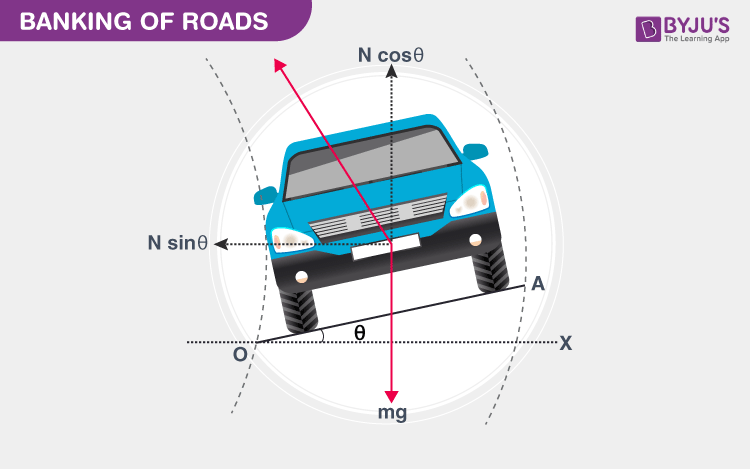## What Is Banking of Roads?

Banking of roads is defined as the phenomenon in which the outer edges are raised for the curved roads above the inner edge to provide the necessary centripetal force to the vehicles so that they take a safe turn. Now, let us recall, what is centripetal force? It is the force that pulls or pushes an object toward the center of a circle as it travels, causing angular or circular motion. In the next few sections, let us discuss the angle of banking and the terminologies used in the banking of roads.

Another terminology used is banked turn which is defined as the turn or change of direction in which the vehicle inclines towards inside. The angle at which the vehicle is inclined is defined as the bank angle. The inclination happens at the longitudinal and horizontal axis.

Read More: Centripetal and Centrifugal Force### Angle of Banking

Consider a vehicle of mass ‘m’ with moving speed ‘v’ on the banked road with radius ‘r’. Let ϴ be the angle of banking, with frictional force f acting between the road and the tyres of the vehicle.

Total upwards force = Total downward force

NcosΘ =mg+fsinΘ

Where,

NcosΘ: one of the components of normal reaction along the verticle axis

mg: weight of the vehicle acting vertically downward

fsinΘ: one of the components of frictional force along the verticle axis

therefore, mg = NcosΘ – fsinΘ (eq.1)

mv2/r = NsinΘ +fcosΘ (eq.2)

Where,

NsinΘ: one of the components of normal reaction along the horizontal axis

fcosΘ: one of the components of frictional force along the horizontal axis

$$\begin{array}{l}\frac{\frac{mv^{2}}{r}}{mg}=\frac{Nsin\Theta +fcos\Theta }{Ncos\Theta -fsin\Theta }\end{array}$$
(after diving eq.1 and eq.2)

therefore,

$$\begin{array}{l}\frac{v^{2}}{rg}=\frac{Nsin\Theta +fcos\Theta }{Ncos\Theta -fsin\Theta }\end{array}$$

Frictional force

$$\begin{array}{l}f=\mu _{s}N\end{array}$$
$$\begin{array}{l}\frac{v^{2}}{rg}=\frac{Nsin\Theta +\mu _{s}Ncos\Theta }{Ncos\Theta -\mu _{s}Nsin\Theta }\end{array}$$
$$\begin{array}{l}\frac{v^{2}}{rg}=\frac{N(sin\Theta +\mu _{s}cos\Theta )}{N(cos\Theta -\mu _{s}sin\Theta )}\end{array}$$
$$\begin{array}{l}\frac{v^{2}}{rg}=\frac{(sin\Theta +\mu _{s}cos\Theta )}{(cos\Theta -\mu _{s}sin\Theta )}\end{array}$$
$$\begin{array}{l}\frac{v^{2}}{rg}=\frac{(tan\Theta +\mu _{s})}{(1-\mu _{s}tan\Theta )}\end{array}$$

therefore,

$$\begin{array}{l}v=\sqrt{\frac{rg(tan\Theta +\mu _{s})}{(1-\mu _{s}tan\Theta )}}\end{array}$$
$$\begin{array}{l}v_{max}=\sqrt{rg tan\Theta }\end{array}$$
$$\begin{array}{l}tan\Theta =\frac{v^{2}}{rg}\end{array}$$
$$\begin{array}{l}\Theta =tan^{-1}\frac{v^{2}}{rg}\end{array}$$

Above is the expression for the angle of banking.

Related Physics Articles:

## What keeps the moon around the Earth?To know more about other Physics related concepts, stay tuned with BYJU’S – The Learning app!